This article I have explain how to used sum function, when working with excel. For example calculate student mark, product total price or total quantity. Sum formula used with many condition that described one by one below with example.

##### Sum

First create your excelling sheet and insert some dummy records as below.

##### Figure 1: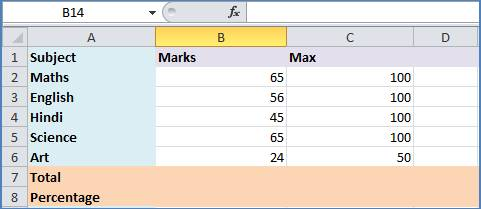Now, Select cell where you see populated value then go to formula bar and used below formula for sum and press enter button.

=SUM (B2:B6)

##### Figure 2:See out put

##### Figure 3:Same formula you can apply for total. For example, if you want to calculate some of multiple columns or multiple row value, then simple pass cell index range. For example if I want get total value of “Marks” and “Max” column then formula as given below

=SUM (B2:C6)

##### Sum a range of cells OFFSET

If you insert a row directly above the SUM function in the previous example, the new row may not be included in the SUM. It may continue to sum cells B2:B6, and ignore B7. To ensure that new rows are included in the total, you can use the OFFSET function with the SUM function.

Formula is given below

=SUM (B2:OFFSET(B7,-1,0))

Now insert one row and see output

##### Figure 4: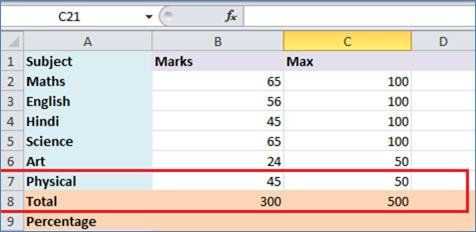You have seen, when new row added into sheet, that value auto calculated into in to “Total” row.

##### Sum cells that match criteria – SUMIF

You can calculate a total for rows that meet a specific criterion. In this example only the rows with Laptop orders will be included in the total. Below I have given formula and prefer to write don’t copy past.

=SUMIF(A2:A9,"Laptop",B2:B9)

##### Figure 5: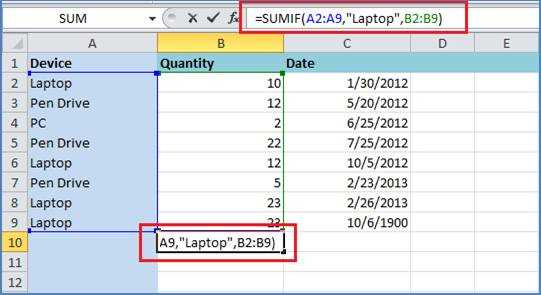Match criterion in a string

You can add cells that contain a criterion as part of the cell's contents. For example all Laptop, Dell Laptop, and HCL Laptop etc. orders will be summed, because they contain the string "Laptop".

=SUMIF(A2:A9,"*Laptop*",B2:B9)

##### Figure 6:##### Criterion and operator

You can use an operator with a criterion. In this example only the rows where the number of sales reps is greater than or equal to ten will be included in the total.

=SUMIF(B2:B9,">=10",C2:C9)

##### Figure 7:##### Sum cells that match multiple criteria - SUMIFS

In Excel 2007 and later versions, you can use the SUMIFS function to calculate a total for rows that meet two or more criteria. In this example only the row where the Device is "Laptop" and the number of order is greater than or equal to ten will be included in the min order.

=SUMIFS(B2:B9,A2:A9,"*LAPTOP*",C2:C9,">=10")

##### Figure 8: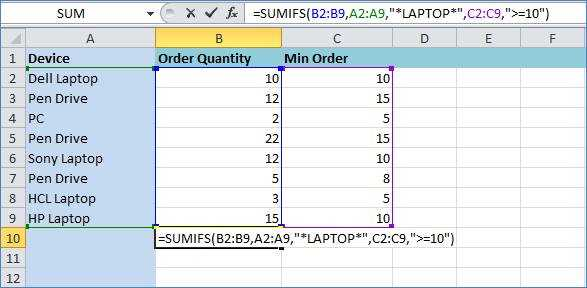##### Sum of Few Top Numbers

If a few numbers are to be summed, e.g. top 3, you can type the numbers into the formula. For example:

=SUM(LARGE(C2:C11,{1,2,3}))

##### Figure 9: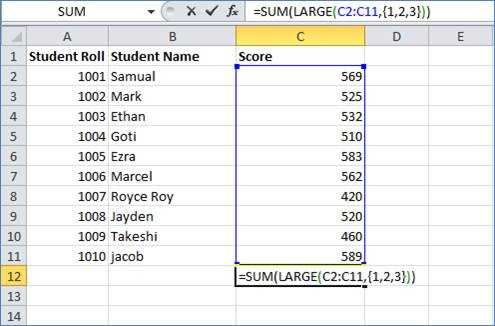##### Sum of Many Top Numbers

If many top numbers are to be summed you can include the INDIRECT function in the formula with the SUM function. In the INDIRECT function, use row numbers that represent the numbers you want to include. In this example, rows 1:10 are used, so the top 5 numbers in the referenced range will be summed.

=SUM(LARGE(C2:C11,ROW(INDIRECT("1:5"))))

Note: This is an array formula, and must be array-entered. To do this, hold the Ctrl and Shift keys, and press Enter

##### Figure 10: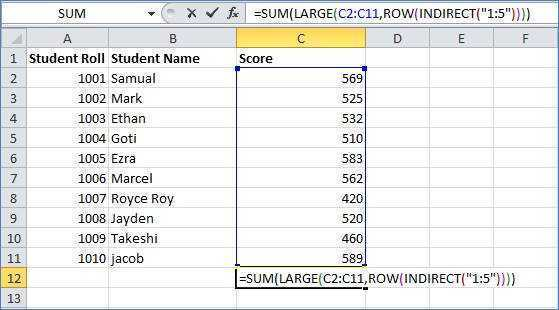##### Sum Variable Top Numbers

If a variable number of top numbers are to be summed you can include the INDIRECT function in the formula with the SUM function, as shown above, and refer to a cell that holds the variable..

In cell d2, type the number of top cells, e.g. 5

=SUM(LARGE(C2:C11,ROW(INDIRECT("1:"&D2))))

This is an array formula, and must be array-entered. To do this, hold the Ctrl and Shift keys, and press Enter

##### Figure 11:Modified On Nov-30-2017 01:28:43 AM